Disclosure: This post may contain affiliate links, meaning when you click the links and make a purchase, we receive a commission.

# Excel Dynamic Range Based on Cell Value

In this article, we will be discussing the “Excel Dynamic range Based on Cell Value”. Here, we will be creating dynamic ranges without selecting them based on some cell value. We will be performing some calculations to see how his thing actually works.

## Using the INDIRECT & CONCATENATE Function to create Dynamic Range

Let`s say we have a column containing some numbers. We want to perform a sum calculation based on a cell value. In a specific cell of our worksheet, we will be indicating the number of cells of that specified column. Based on the cell value the calculation will be done. Like, if we write 2 in that specific cell, the formula which we will be using will calculate the sum of the 1st two cells of the column. We will be using the INDIRECT and CONCATENATE functions together. The procedure is shown below.

• First, in column B put some numbers. Make two heading Cell Value & SUM in Cell C1 and Cell D1 accordingly.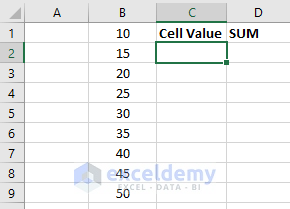• IN C2 type a number say 4 and type this below formula in D2: `=SUM(B1:INDIRECT(CONCATENATE("B",C2)))`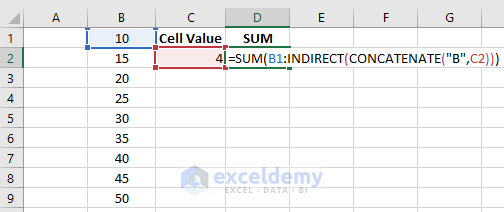• After pressing Enter you will see the below result.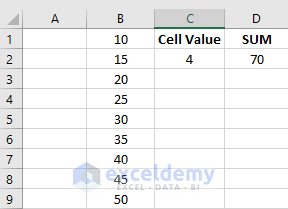• Here the formula calculates the sum of the first 4 cells of column B. By changing the value of cell C2, the result will also change.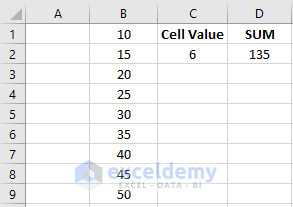Note: If the cell value (C2) is greater than the number of cells of column (B), the formula will take the total number of cells of the column every time while doing the calculation. This is because the vacant cell of Column B has no values. Whenever you refer a cell value which is larger than the column size, the formula will always take the total number of cells containing numbers in a column.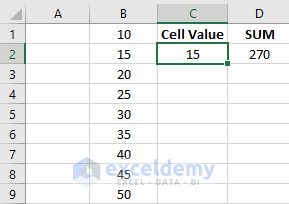## Choosing Cells Dynamically in a Column Based on Two cell Values (Using INDIRECT Function)

This example is similar to the previous one. The only difference here is that we will be choosing the range of the column B based on 2 cell values. The cell C2 will select the first cell of the dynamic range and cell C3 will define the last cell of the range. We will be performing the same calculation. The formula will be different and will not contain the CONCATENATE function. The process is shown below.

• First, in column B put some numbers. Make two heading Cell Value & SUM in Cell C1 and Cell D1 accordingly.• IN C2 type 2 and in C3 type 6. Type the formula `=SUM(INDIRECT("B"&C2&":B"&C3))` in cell D2.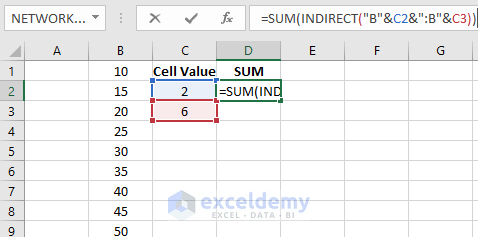• After pressing Enter you will see the below result.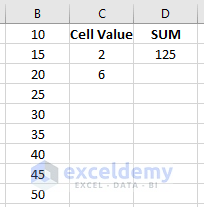• Here the formula calculates the number from cell B2 to B6. By changing the numbers, you will see the result changes.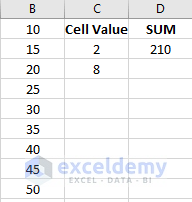## Choosing Cells Dynamically Based on Two cell Values (Using OFFSET Function)

The OFFSET function can be used to make a range of cells dynamic. Here we will select the number of cells and columns separately to perform the sum calculation. The procedure is given below.

• Let`s say we have 2 set of numbers in Column B and Column C. Make 3 headers titled as Cell Value, Column, and SUM.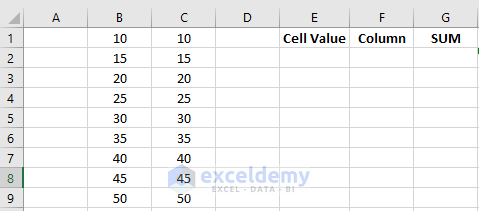• In E2 type 7 and in F2 type 2. Write down the formula `=SUM(OFFSET(B1,0,0,E2,F2))` in cell G2 and press Enter.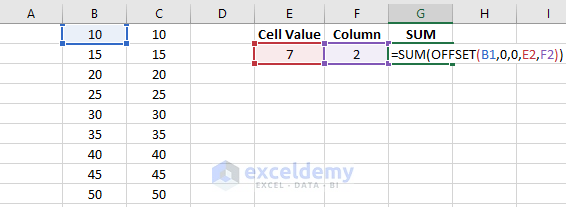• After pressing Enter you will get to see the below result.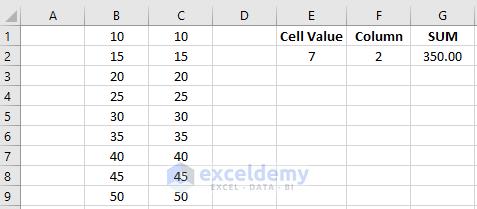• Here the formula first starts the calculation from B1 and comes to cell B7 because the height of the offset function is given as E2 which contains the value 7. As the width is given as 2, so the whole range of calculation will be B1:C7.

## Conclusion

In this article, we analyzed Excel dynamic range. We create the dynamic ranges using some formulas. The calculation of those dynamic ranges was done based on some cell values. Hope you will love this article.

Stay tuned for more useful articles.

Hello!

Welcome to my Profile. Here I will be posting articles related to Microsoft Excel. I have completed my BSc in Electrical and Electronic Engineering from American International University-Bangladesh. I am a diligent, goal-oriented engineer with an immense thirst for knowledge and attitude to grow continuously. Continuous improvement and life-long learning is my motto.

We will be happy to hear your thoughts